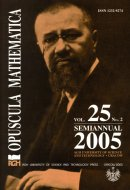Opuscula Math. 25, no. 2 (2005), 325-332

Opuscula Mathematica

# The exact values of nonsquare constants for a class of Orlicz spaces

Jincai Wang

Abstract. We extend the $$M_{\triangle}$$-condition from [Han J.,Li X.: On Exact Value of Packing for a Class of Orlicz Spaces. (Chinese), Journal of Tongji Univ. 30 (2002) 7, 895–899] and introduce the $$\Phi_{\triangle}$$-condition at zero. Next we discuss nonsquare constant in Orlicz spaces generated by an $$N$$-function $$\Phi(u)$$ which satisfy $$\Phi_{\triangle}$$-condition. We obtain exact value of nonsquare constant in this class of Orlicz spaces equipped with the Luxemburg norm.

Keywords: nonsquare constant, Orlicz space, $$\Phi_{\triangle}$$-condition.

Mathematics Subject Classification: 46B20, 46E30.

Full text (pdf)

• Jincai Wang
• Suzhou University, Department of Math., Suzhou, 215006 P. R. China